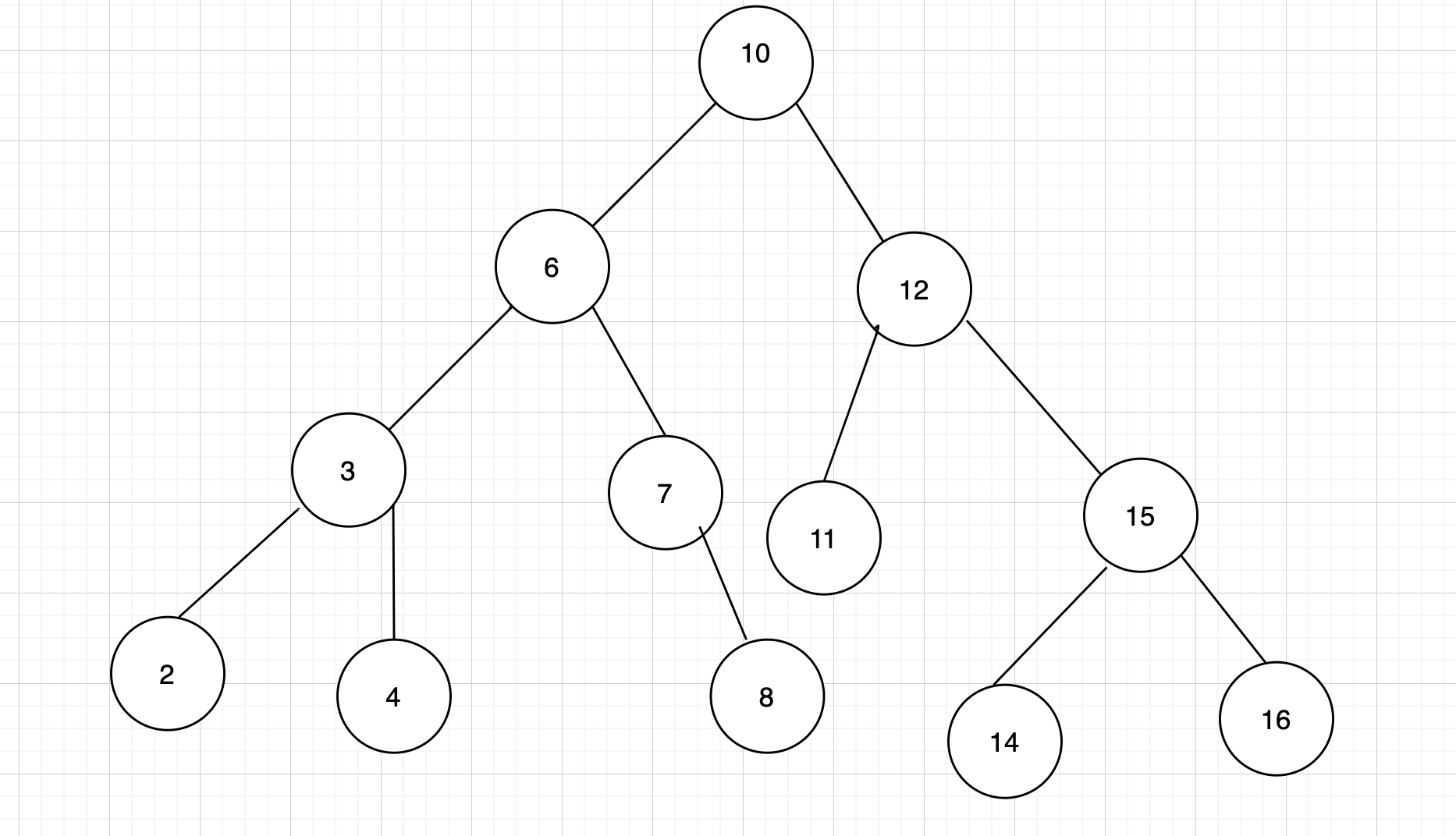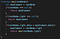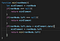# Finding Maximum and Minimum Element in a Binary Search Tree

First let’s get a recap of what are trees.

Trees are data structure that have many children. The root node is the top most node in the tree and the child node emerge from the root node.

Now what are binary trees?

Binary trees are tree data structure that have only two child(left and right) hence the name and the nodes which does not have any children are leaf nodes.The left and right child or nodes are subtrees off binary tree.

Binary search trees are binary trees but the left half /subtree of a binary search tree is always lesser than root node and the right half/subtree of a binary search tree is always greater than the root node.The left subtree and the right subtree must also be a binary search tree and there most be no duplicate nodes.Binary Search Tree

Finding the maximum element in a binary tree:

• First we declare a variable maxElement and assign the root node to it(not the data/value of the root node but the root node) since we have to return the maximum element and not the data/value of the maximum element.
• Next we have to see the value of the root node and if the root node is null that means it does not exist we return the root node since it is clear that the binary search tree is empty because it does not contain any root node.
• If however there is a root node, then we check on the right since the values greater than the root nodes can only exist on the right. However if this was a binary tree but not a binary search tree, then we would have to check on both left and right side of the tree.
• Next we go to the right and if the value of the right of the root node is null then that means there is no right element or node in the tree , we return the root node since that is the maximum element in the tree.
• If however the tree does have a right side, then we check the data inside the maxElement and if it is less than the data inside the right of the root node , then we assign the right of the root node to the maxElement and using recursion we traverse the right half of the tree by passing the right of the node we are in inside the recursive function until the right hits null and then we hit the base case and return the element.Finding Maximum Element in a Binary search Tree

For finding the minimum element in a binary search tree we follow the same steps except for traversing to the right we traverse left since the elements or nodes to the left of the tree are lesser than the root node.

Finding the minimum element in a binary search tree:

• First we we declare a variable minElement and assign the root node to it(not the data/value of the root node but the root node) since we have to return the minimum element and not the data/value of the minimum element.
• we have to check the value of the root node and if the root node is null then the tree is empty and we return the root node.
• If however there is a root node, we check the left of the root node and if the left of the root node is null, then it means that the tree has no left nodes and the minimum element or node is the root node and we return it.
• If the tree has a left node , we check if the data inside the minElement is less than the data inside the left node and if it is then we replace the previous value of the minElement with the new value or new element and we keep traversing the tree using recursion by passing the left of the current node inside the function and keep replacing the element in the minElement until we reach the left most node of the tree and hit null , that this node does not have any child i.e a leaf node and hit the base case and return the minimum element.Finding Minimum Element in a Binary Search Tree

Resources:

https://www.geeksforgeeks.org/difference-between-binary-tree-and-binary-search-tree/

https://www.youtube.com/watch?v=w8lxL-ZTHKs

Flatiron School Alumni | Software Engineer

## More from Arpita Dutta

Flatiron School Alumni | Software Engineer